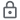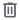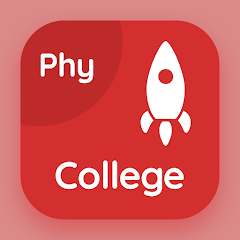# College Physics Quiz

1K+Everyone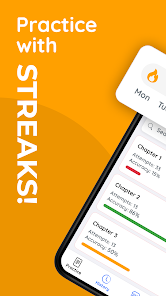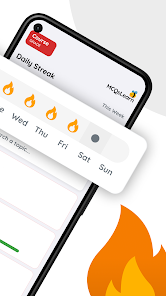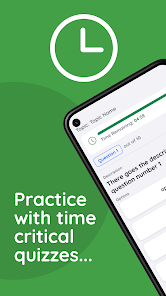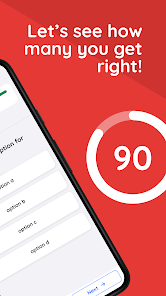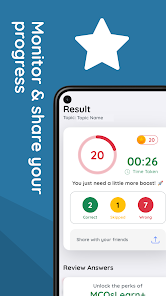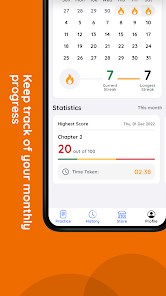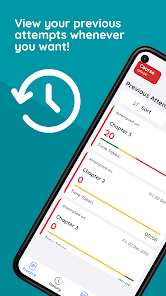College Physics App:

College physics quiz app with free download to install "Physics Quiz" app (Android) to practice hundreds of physics quiz based MCQs. "College Physics" app download with trivia questions and answers, college level physics MCQs to solve self-assessment tests. "College Physics Quiz" app, textbook revision notes helps to practice test questions for beginners and advanced level students on Android smartphones.

Complete college physics app, a textbook application with IGCSE/NEET/MCAT/MDCAT/SAT/ACT preparation mock tests. "College Physics Notes" app is a study guide for students, beginners and advanced level learning from physics textbook topics as:

Chapter 1: Motion and force quiz
Chapter 2: Work and energy quiz
Chapter 3: Atomic spectra quiz
Chapter 4: Circular motion quiz
Chapter 5: Current and electricity quiz
Chapter 6: Electromagnetic induction quiz
Chapter 7: Electromagnetism quiz
Chapter 8: Electronics quiz
Chapter 9: Electrostatic quiz
Chapter 10: Fluid dynamics quiz
Chapter 11: Measurements in physics quiz
Chapter 12: Modern physics quiz
Chapter 13: Vector and equilibrium quiz

Solve "Work and Energy Quiz" app download to practice test questions: Energy, conservation of energy, non-conventional energy sources, work done by a constant force, work done formula, physics problems, and power.

Solve "Atomic Spectra Quiz" app download to practice test questions: Bohr's atomic model, electromagnetic spectrum, inner shell transitions, and laser.

Solve "Circular Motion Quiz" app download to practice test questions: Angular velocity, linear velocity, angular acceleration, angular displacement, law of conservation of angular momentum, artificial gravity, artificial satellites, centripetal force (CF), communication satellites, geostationary orbits, moment of inertia, orbital velocity, angular momentum, rotational kinetic energy, and weightlessness in satellites.

Solve "Current and Electricity Quiz" app download to practice test questions: Current and electricity, current source, electric current, carbon resistances color code, potential difference, Kirchhoff's law, ohms law, power dissipation, resistance and resistivity, and Wheatstone bridge.

Solve "Electromagnetic Induction Quiz" app download to practice test questions: Electromagnetic induction, AC and DC generator, EMF, induced current, induction, and transformers.

Solve "Electromagnetism Quiz" app download to practice test questions: Electromagnetism, Ampere's law, cathode ray oscilloscope, e/m experiment, force on moving charge, galvanometer, magnetic field, and magnetic flux density.

Solve "Electronics Quiz" app download to practice test questions: Electronics, logic gates, operational amplifier (OA), PN junction, rectification, and transistor.

Solve "Electrostatic Quiz" app download to practice test questions: Electrostatics, electric field lines, electric flux, electric potential, capacitor, Coulomb's law, Gauss law, electric and gravitational forces, electron volt, and Millikan experiment.

Solve "Fluid Dynamics Quiz" app download to practice test questions: Applications of Bernoulli's equation, Bernoulli's equation, equation of continuity, fluid flow, terminal velocity, viscosity of liquids, viscous drag, and Stroke's law.

Solve "Modern Physics Quiz" app download to practice test questions: Modern physics, and special theory of relativity.

Solve "Vector and Equilibrium Quiz" app download to practice test questions: Vectors, vector concepts, vector magnitude, cross product of two vectors, vector addition by rectangular components, product of two vectors, equilibrium of forces, equilibrium of torque, product of two vectors, solving physics problem, and torque.

"College Physics MCQs" app helps to solve physics Multiple Choice Questions (MCQs) from every chapter, comparing with answer key after every 10 random trivia quiz questions.

Looking forward to provide best user experience through college physics application!
Updated on
Nov 23, 2023

## Data safety

Safety starts with understanding how developers collect and share your data. Data privacy and security practices may vary based on your use, region, and age. The developer provided this information and may update it over time.No data shared with third parties